# Compatible pair of actions

(diff) ← Older revision | Latest revision (diff) | Newer revision → (diff)

## Definition

### Definition with left action convention

Suppose$G$ and$H$ are groups. Suppose$\alpha:G \to \operatorname{Aut}(H)$ is a homomorphism of groups, defining a group action of$G$ on$H$. Suppose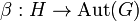$\beta:H \to \operatorname{Aut}(G)$ is a homomorphism of groups, defining a group action of$H$ on$G$. For$g \in G$, denote by$c_g: G \to G$ the conjugation map by$g$. See group acts as automorphisms by conjugation. Then, we say that the actions$\alpha,\beta$ form a compatible pair if both these conditions hold:

•$\beta(\alpha(g_1)(h))(g_2) = c_{g_1}(\beta(h)(c_{g_1^{-1}}(g_2)))) \ \forall \ g_1,g_2 \in G, h \in H$
•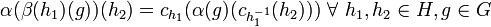$\alpha(\beta(h_1)(g))(h_2) = c_{h_1}(\alpha(g)(c_{h_1^{-1}}(h_2))) \ \forall \ h_1,h_2 \in H, g \in G$

The above expressions are easier to write down if we use$\cdot$ to denote all the actions. In that case, the conditions read:

•$(g_1 \cdot h) \cdot g_2 = g_1 \cdot (h \cdot (g_1^{-1} \cdot g_2)) \ \forall \ g_1,g_2 \in G, h \in H$
•$(h_1 \cdot g) \cdot h_2 = h_1 \cdot (g \cdot (h_1^{-1} \cdot h_2)) \ \forall \ h_1,h_2 \in H, g \in G$

Here is an equivalent formulation of these two conditions that is more convenient:

•$c_{g_1}(\beta(h)g_2) = \beta(\alpha(g_1)h)(c_{g_1}(g_2)) \ \forall \ g_1,g_2 \in G, h \in H$ (the$g_2$ here is the$c_{g_1^{-1}}g_2$ of the preceding formulation).
•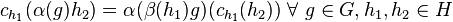$c_{h_1}(\alpha(g)h_2) = \alpha(\beta(h_1)g)(c_{h_1}(h_2)) \ \forall \ g \in G, h_1, h_2 \in H$ (the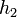$h_2$ here is the$c_{h_1^{-1}}h_2$ of the preceding formulation)

In the$\cdot$ notation, these become:

•$g_1 \cdot (h \cdot g_2) = (g_1 \cdot h) \cdot (g_1 \cdot g_2) \ \forall \ g_1,g_2 \in G, h \in H$ (the$g_2$ here is the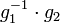$g_1^{-1} \cdot g_2$ of the preceding formulation).
•$h_1 \cdot (g \cdot h_2) = (h_1 \cdot g) \cdot (h_1 \cdot h_2) \ \forall g \in G, h_1,h_2 \in H$ (the$h_2$ here is the$h_1^{-1} \cdot h_2$ of the preceding formulation)

### Definition with right action convention

We can give a corresponding definition using the right action convention, but the literature uses the left action convention, so this definition is intended purely as an illustrative exercise. PLACEHOLDER FOR INFORMATION TO BE FILLED IN: [SHOW MORE]

## Symmetry in the definition

Given groups$G$ and$H$ with actions$\alpha:G \to \operatorname{Aut}(H)$ and$\beta:H \to \operatorname{Aut}(G)$,$\alpha$ is compatible with$\beta$ if and only if$\beta$ is compatible with$\alpha$. In other words, the definition of compatibility is symmetric under interchanging the roles of the two groups.

## Particular cases

• Trivial pair of actions is compatible: If both the actions are trivial, i.e., both the homomorphisms$\alpha,\beta$ are trivial maps, then they form a compatible pair.
• Compatible with trivial action iff image centralizes inner automorphisms: Suppose the homomorphism$\alpha$ is trivial. In that case, the homomorphism$\beta$ is compatible with$\alpha$ if and only if the image of$\beta$ in$\operatorname{Aut}(G)$ is in the centralizer$C_{\operatorname{Aut}(G)}(\operatorname{Inn}(G))$.
• Conjugation actions between subgroups that normalize each other are compatible: If$G,H$ are both subgroups of some group$Q$ that normalize each other (i.e., each is contained in the normalizer of the other), and$\alpha,\beta$ are the actions of the groups on each other by conjugation, then they form a compatible pair. Note that in this case, all the actions are just conjugation in$Q$ and checking the conditions simply amounts to checking two words to be equal.